# cs231n - 이해하기 2

cs231n

• http://cs231n.stanford.edu/

이 포스팅은 딥러닝에 대한 기본 지식을 상세히 전달하기보다는 간략한 핵심과 실제 모델 개발에 유용한 팁을 위주로 정리하였습니다.

## Detection and Segmentation

1) semantic segmentation :

• sliding window
• Fully convolutional : labeling class per every pixel
• downsampling and upsampling : how to upsampling(unpooling)
• nearest neighbor
• bed of nails
• max unpooling(remember which element was max)
• Transpose Convolution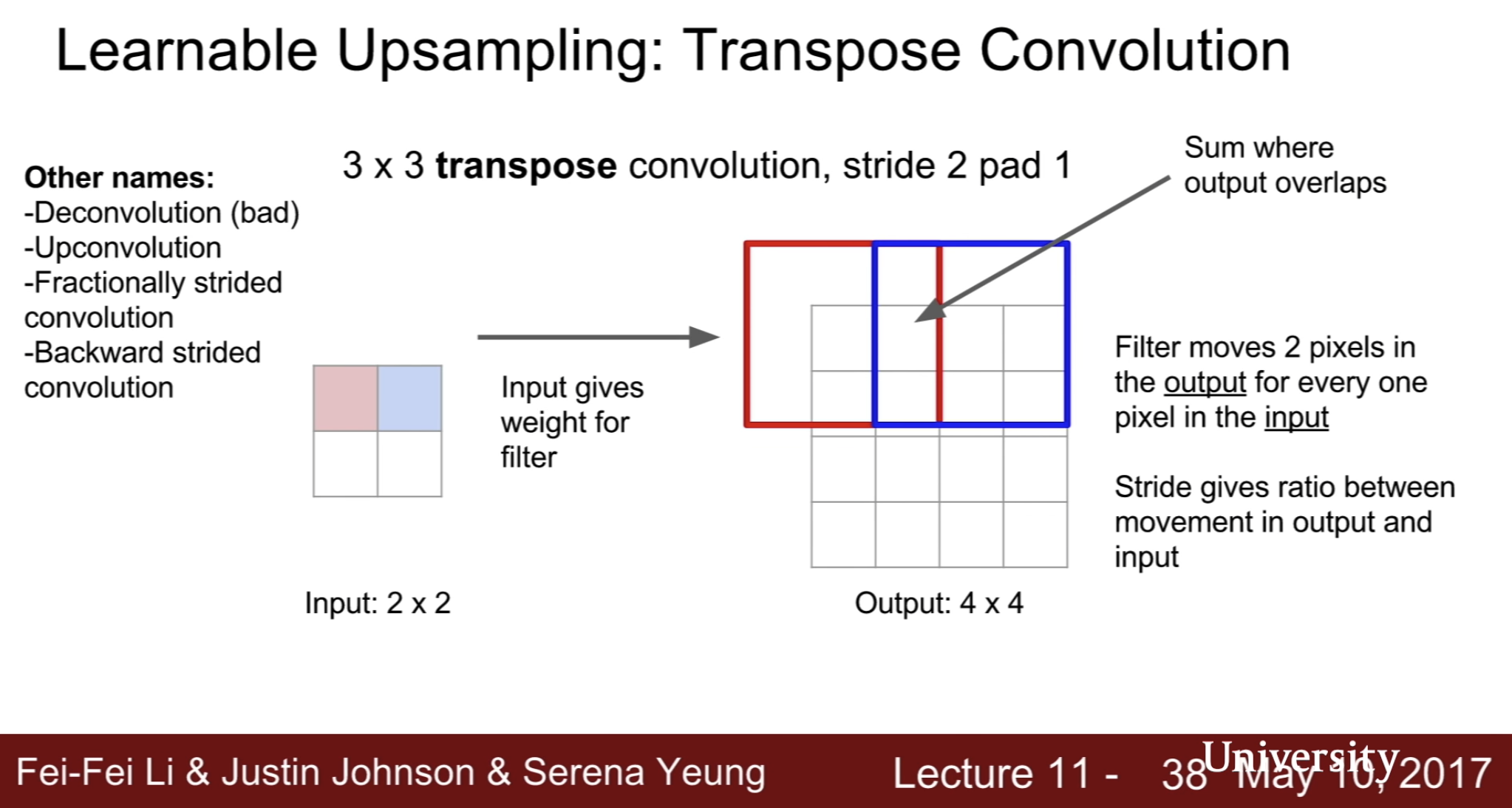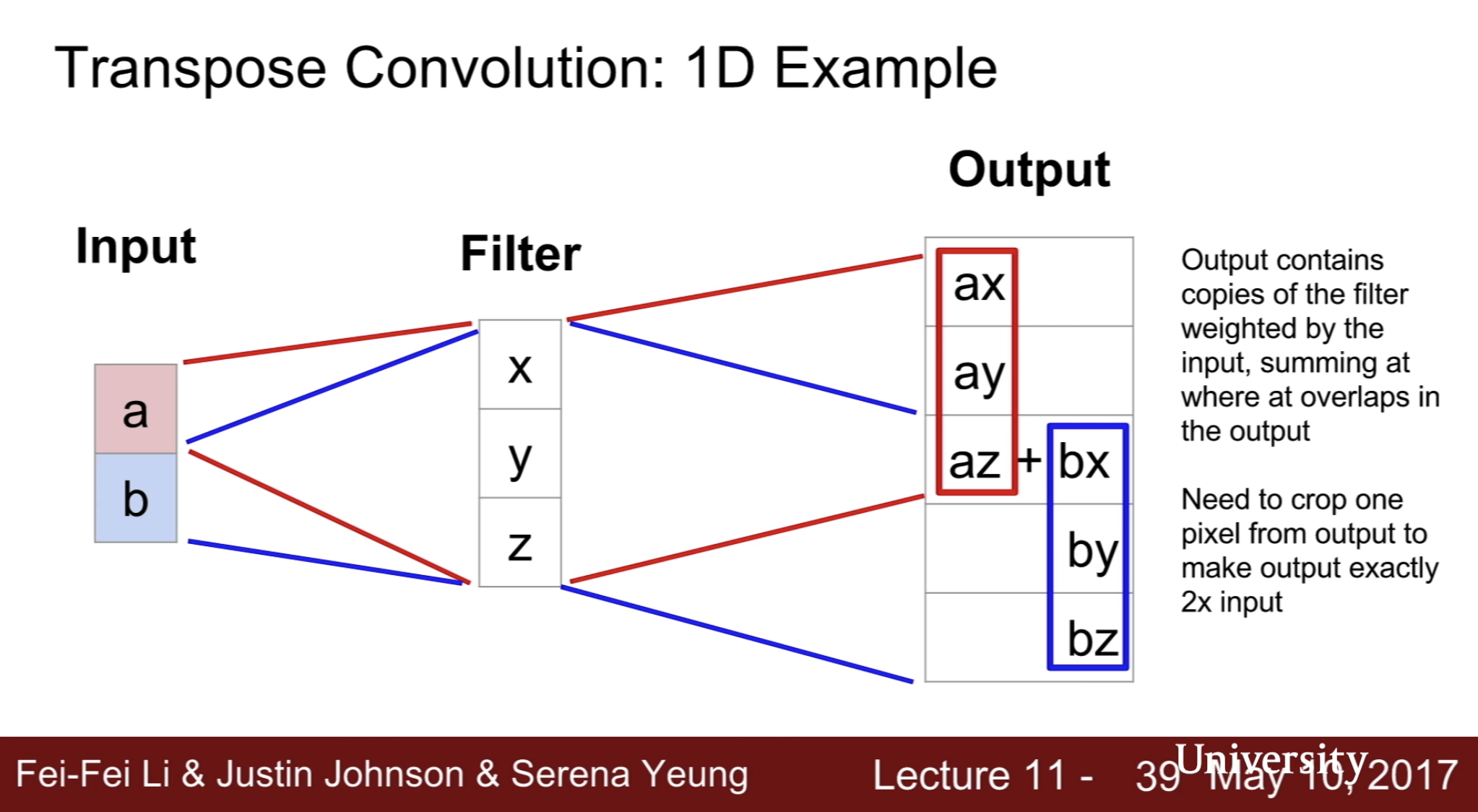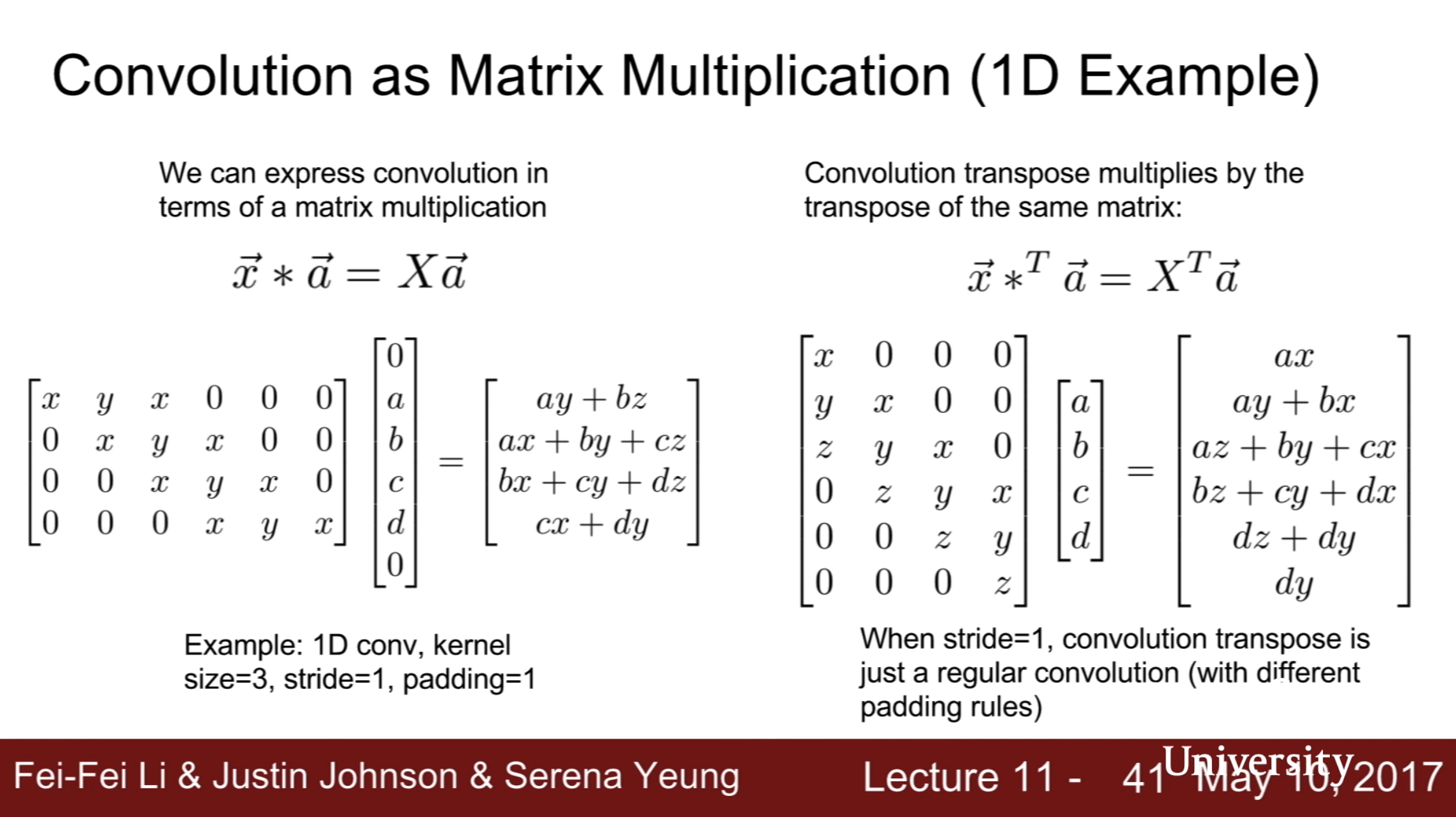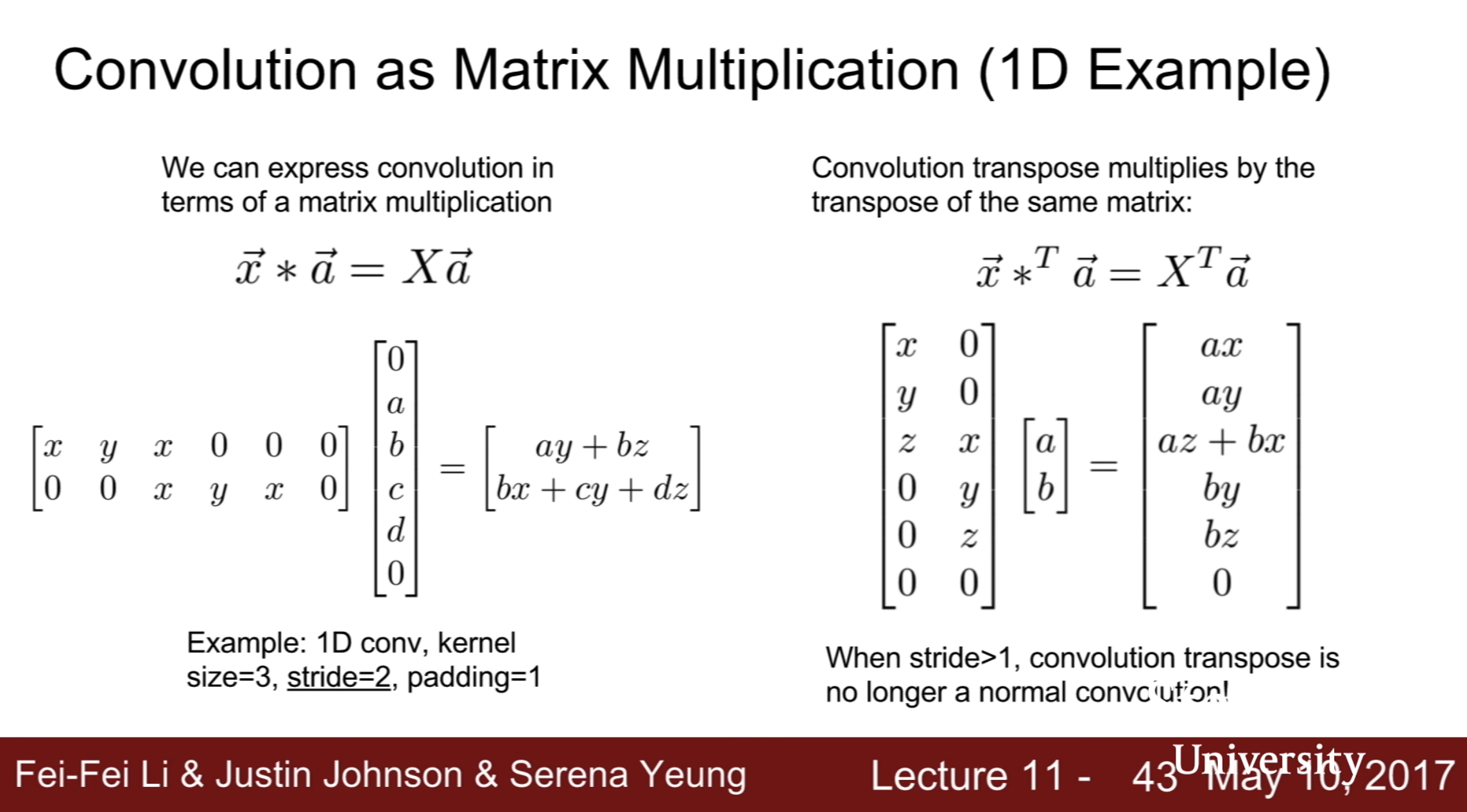2) classification + localization :

• class score : softmax loss
• box coordiantes(x, y, w, h) : L2 loss
• treat localization as a regression problem

multimodal - how to determine weight of two different loss function? loss값 외에 다른 지표를 참고

• aside : Human pose estimation

3) Object Detection

• fixed set of categories and draw box location
• benchmark dataset : PASCAL VOC
• each image needs a different number of outputs - not easy to solve with regression
• sliding window : crops of the image, CNN classifies each crop as object or background
• how to choose crop? need to apply CNN to huge number of locations and scales very expensive
• region proposals : selective search gives 1000 region proposal -> brute force but high recall
• R-CNN
• region of interest(RoI) from a proposal method (~2k)
• Warped image regions
• forward each region through convNet
• classify regions with SVMs
• Box regression

slow train and inference

• fast R-CNN
• Forward whole image through ConvNet
• RoIs from proposal method on convnet feature map of image
• RoI pooling layer
• fully connected
• classification and regression
• faster R-CNN
• make CNN do proposals
• insert region proposal network(RPN) to predict proposals from features
• jointly train with 4 lossess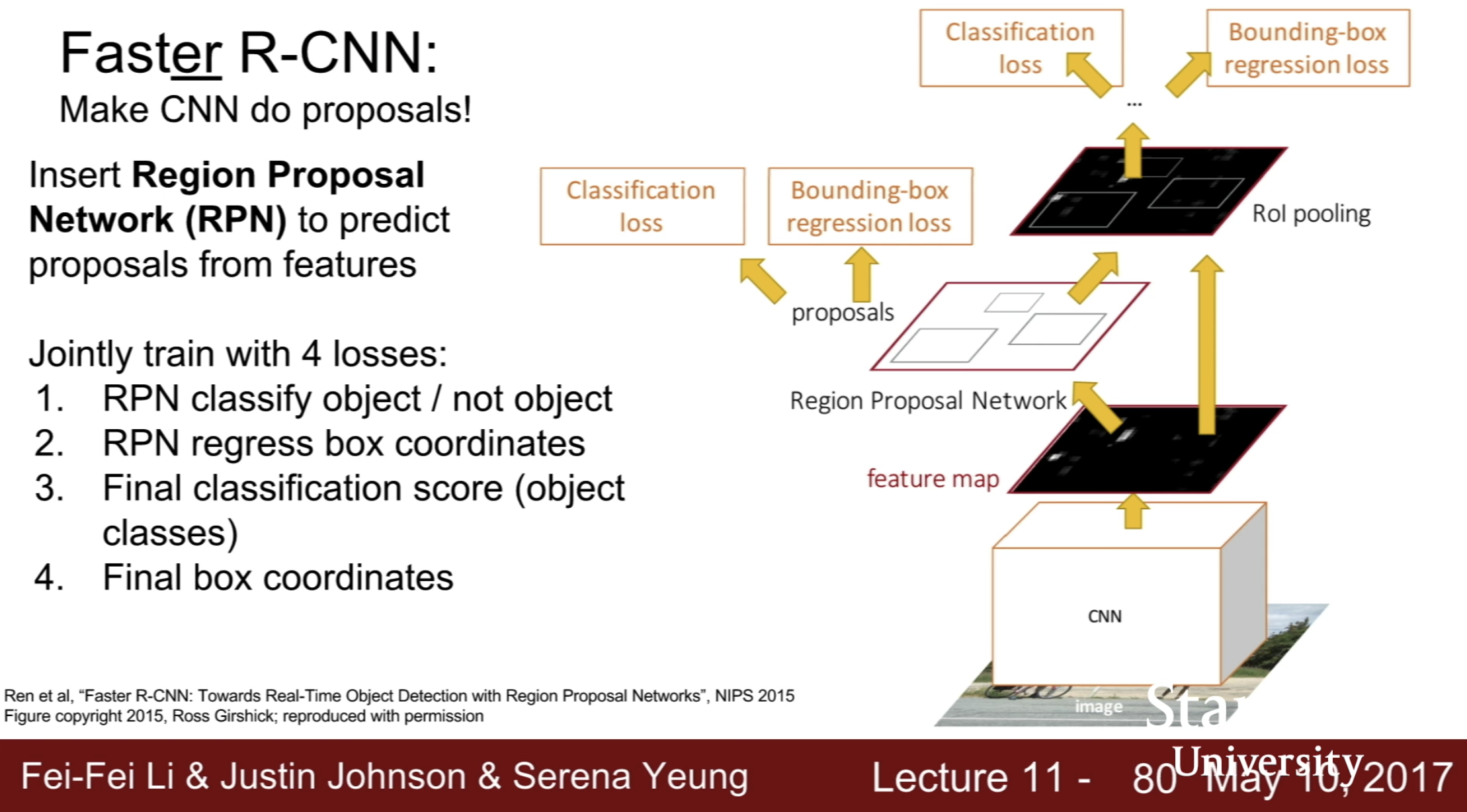• detection without proposals : YOLO / SSD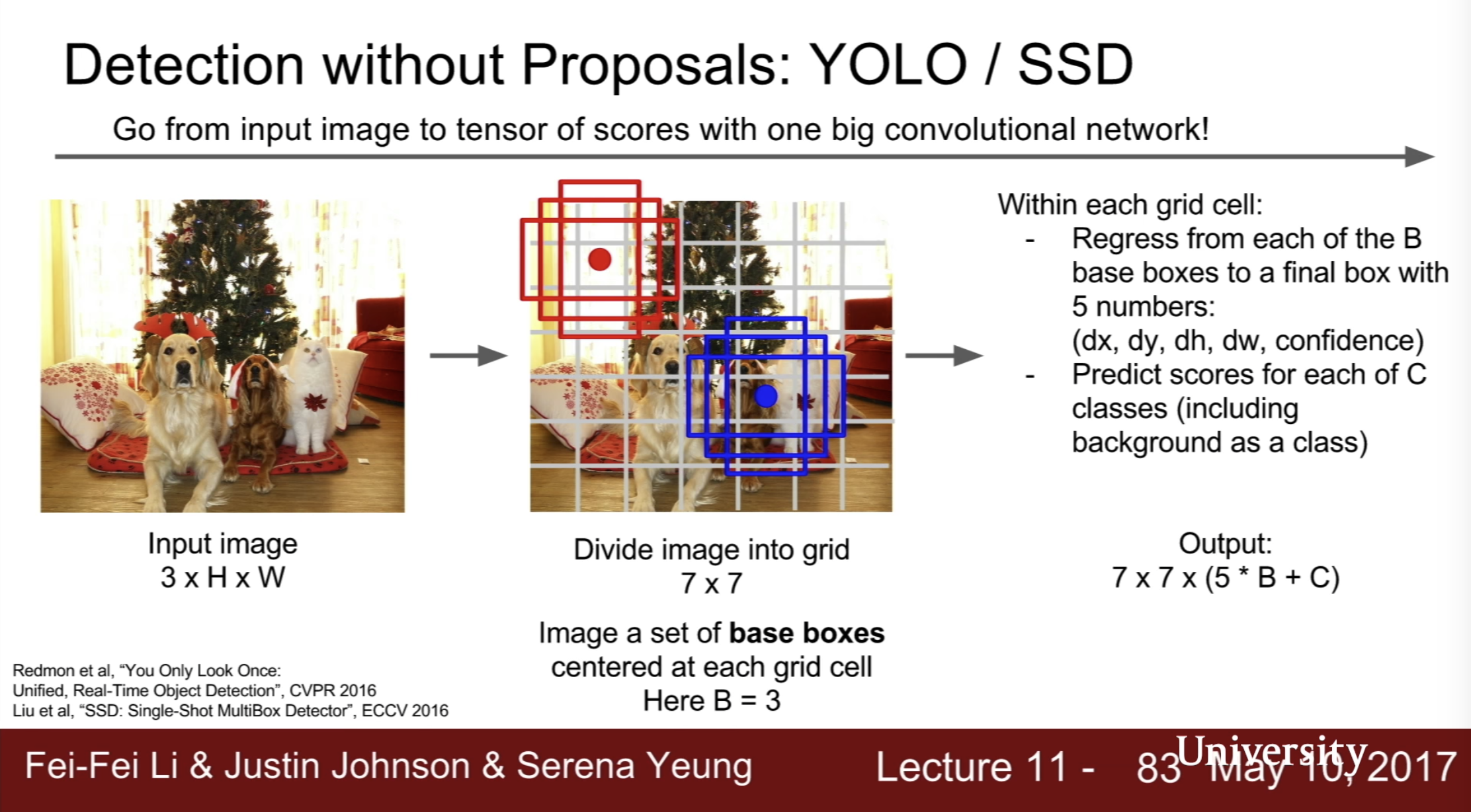4) Instance Segmentation

• Mask R-CNN - similar to faster R-CNN
• can also does pose : add joint coordinates
• bechmark data : microsoft coco data

## Visualizing and Understanding

what’s going on inside ConvNets? What are the intermediate features looking for?

• visualize the filters : raw weights
• not that interesting
• Last Layer :
• check Nearest Neighbors in faeture space(last fc layer)
• dimensionality reduction : PCA, t-SNE
• Occlusion Experiments : 부분적으로 마스크함
• mask한 영역으로 인해 확률이 극격히 변화면 해당 영역은 크리티컬하다고 가정
• saliency maps : 이미지의 각 픽셀들에 대해서 클래스 스코어의 그래디언트를 구함. compute gradient of class score with respect to image pixels
• intermediate feature via guided backprop : which part of image impact to intermediate activation value
• relu : positive gradient만 이용하면 더 나이스 이미지를 얻을수 있다
• gradient ascent : 지금까지는 보통의 백프로파게이션을 통해 이미지의 어떤 부분이 뉴련에 영향을 주는지 알아봤다면(고정된 입력 이미지 값), 그래디언트 어센트는 뉴런의 액티베이션을 최대화하는 방향으로 이미지를 만들어내는 것임(입력 이미지 값을 생성하는 것)
• generate a synthetic image that maximally activates a neuron
• better regrularizer (image prior regualarization)
• optimize in FC6 latent space instead of pixel space
• Fooling Image
• 엘리퍼튼 이미지를 고르고
• 코알라 클래스 스코어를 골라
• 코알라 클래스 스코어를 최대화하도록 이미지를 모디파이
• 네트워크가 코알라로 분류할때까지 반복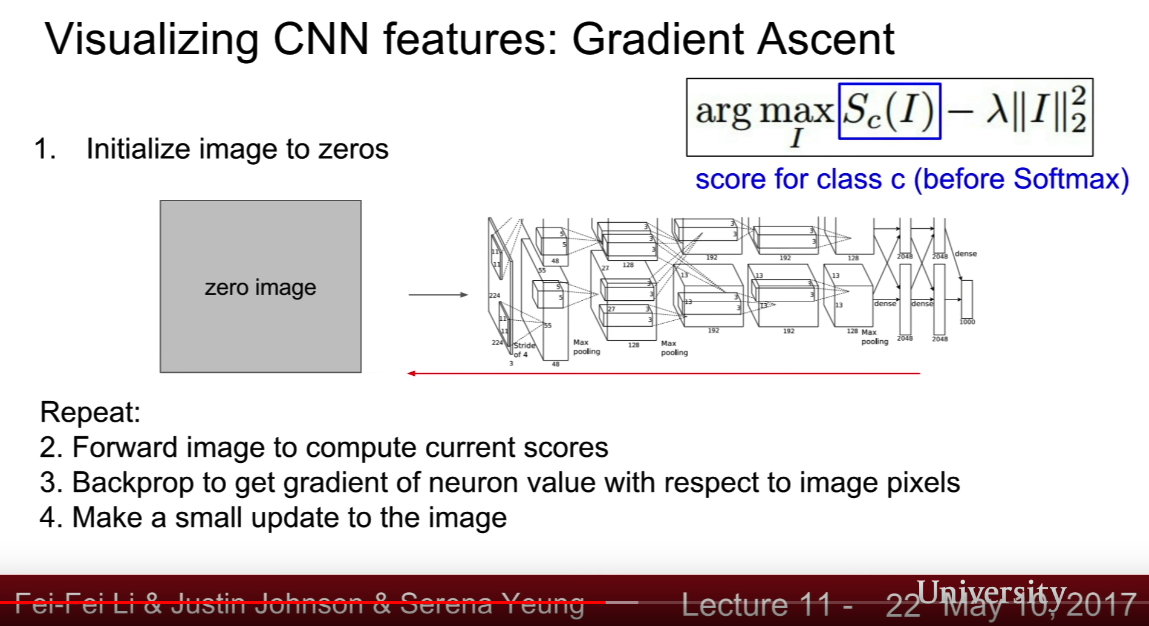• DeepDream
• choose an image and a layer in a CNN : repeat:
• Forward : compute activations at chosen layer
• set gradient of chosen layer equal to its activation
• backward : compute gradient on image
• update image
• Feature Inversion : 피쳐벡터를 뽑고, 그 피쳐벡터에 매칭되는 다른 입풋 이미지를 만들어냄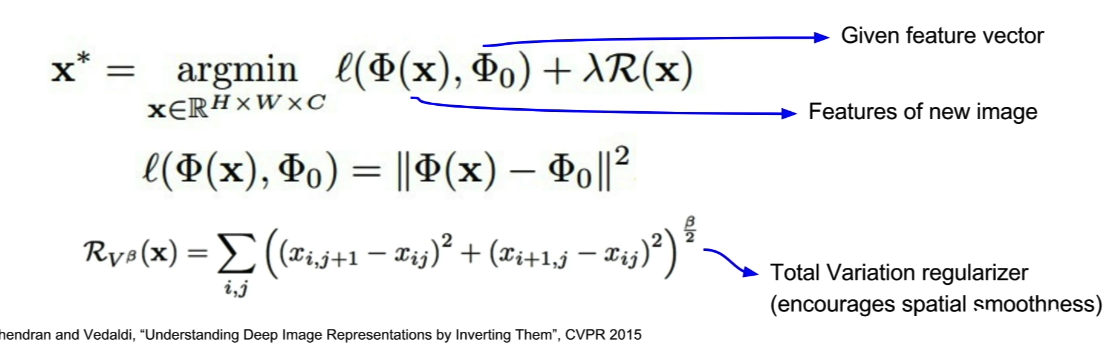• Texture sythesis : given a sample patch of some texture, can we genrate a bigger image of the same texture?
• classical approch : nearest
• neural texture synthesis : gram matrix
• Style Transfer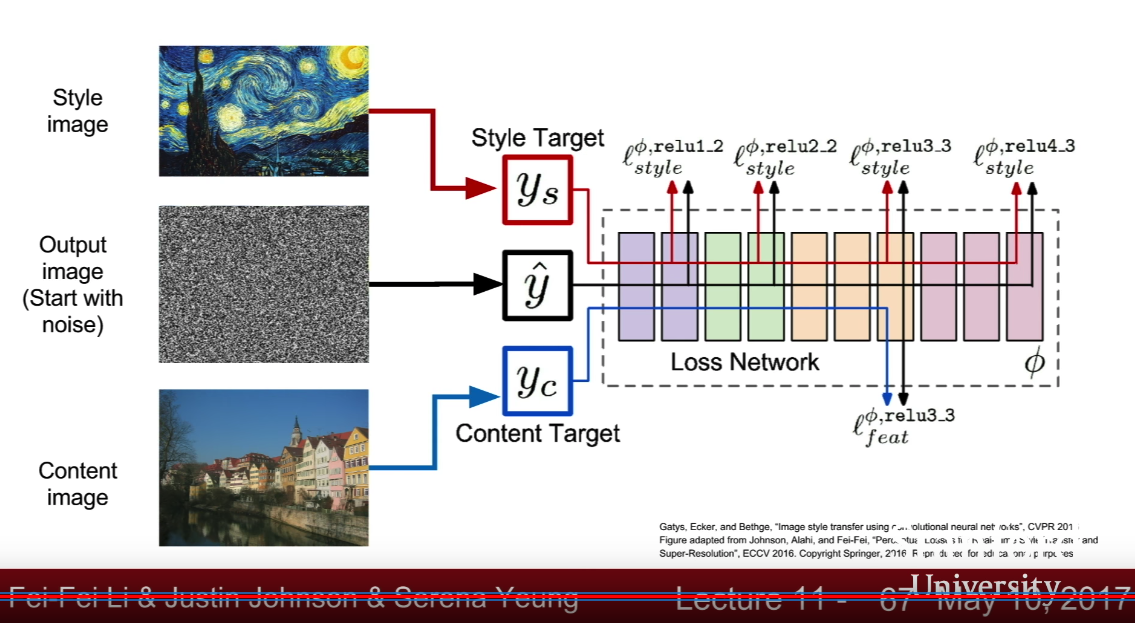• slow : train another model to transfer style
• fast style transfer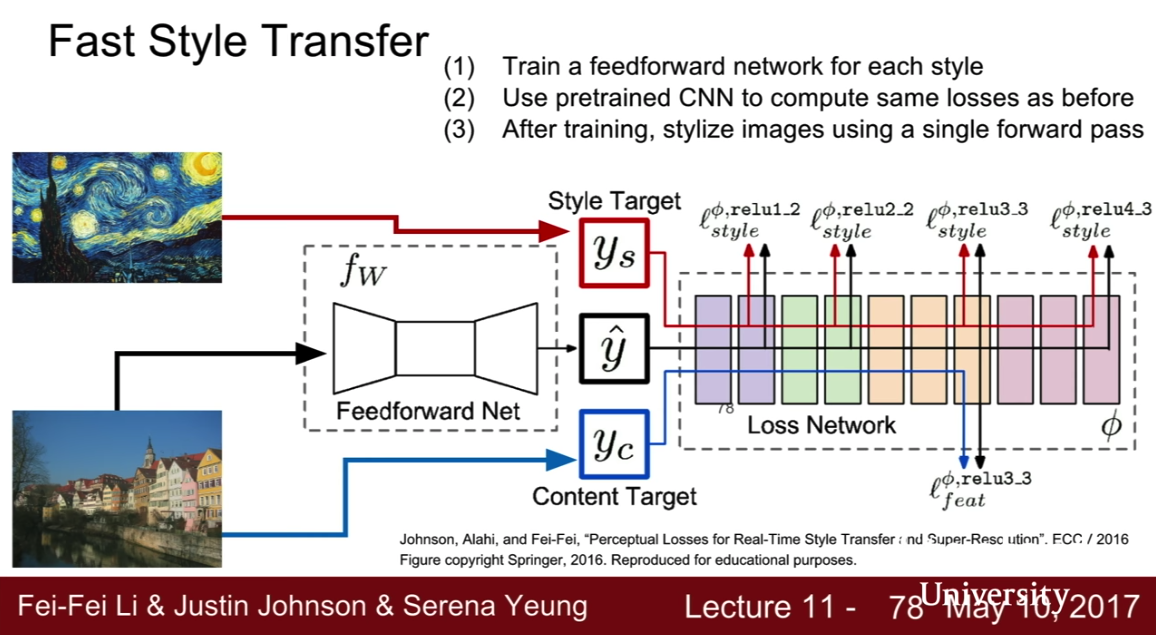## Generative Models

• generative models of time-series data can be used for simulation and planning

• Fully visible belief network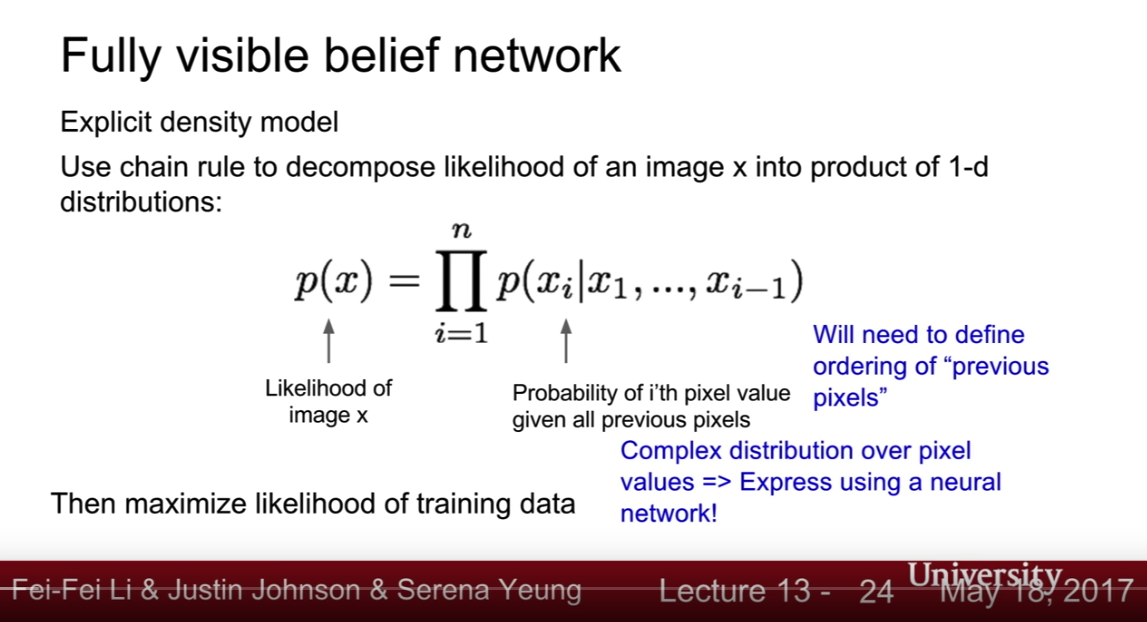• 픽셀의 오더를 어떻게 결정하지? –> pixelRNN

• pixelRNN
• 코너에 있는 픽셀부터 다이어고날 방향으로 시퀄셜로 학습 using RNN (LSTM)
• sequtional is slow
• pixelCNN
• 코너에 있는 픽샐부터 시작하는 것은 같으나
• context region(previous pixels)으로부터 모델링되는 것
• training is faster but generation must still process sequentially
• Variational auto-encoder
• intractible to compute p(x|z) for every z
• in addition to decoder pθ(x|z), define additional encoder qφ(z|x) that approximates pθ(z|x)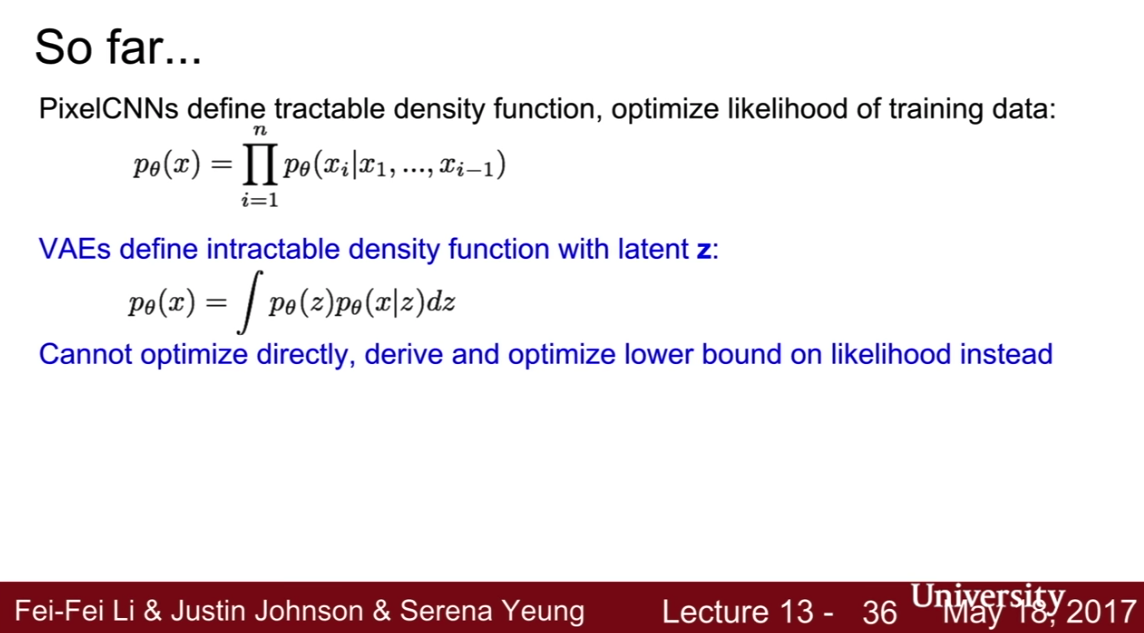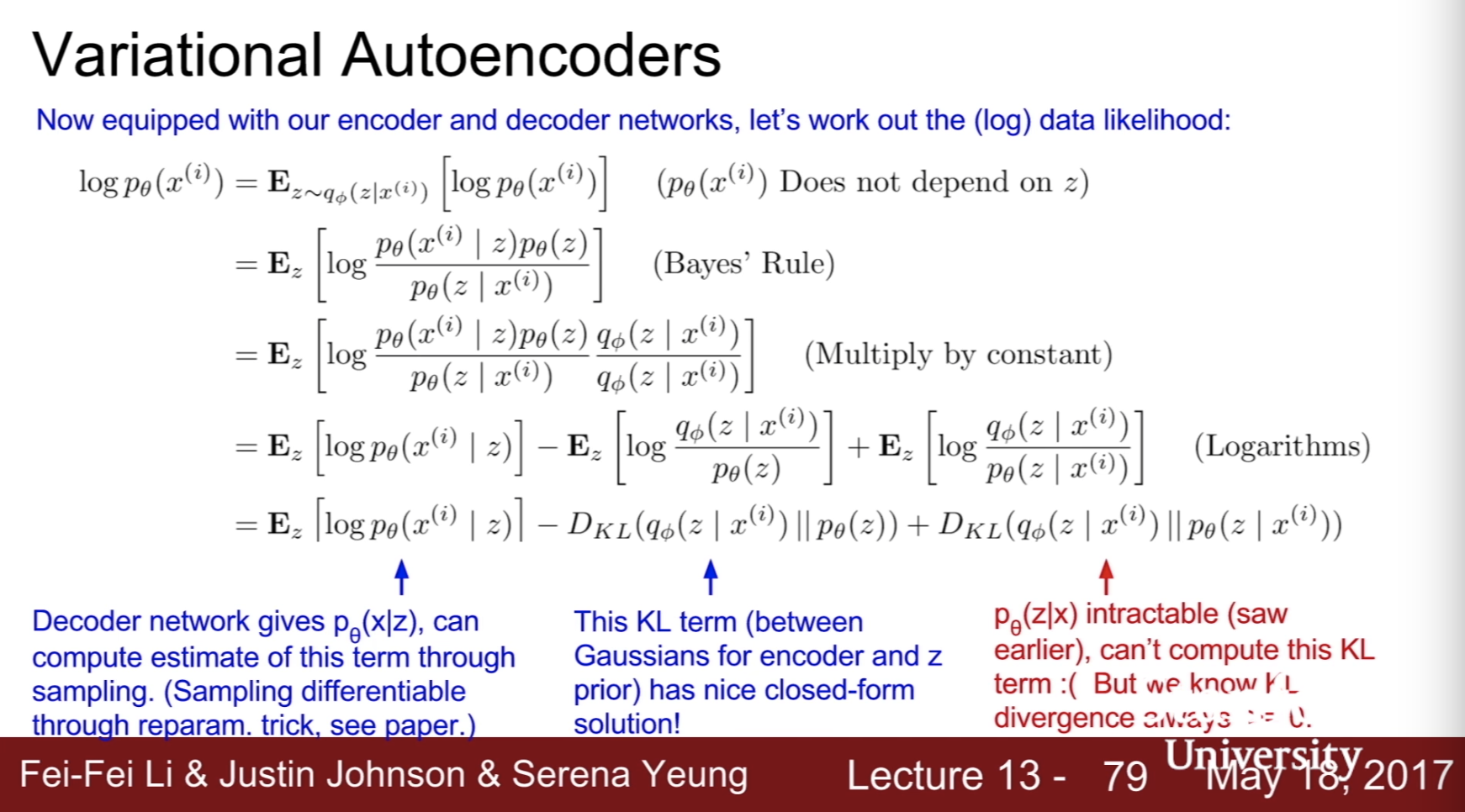• GAN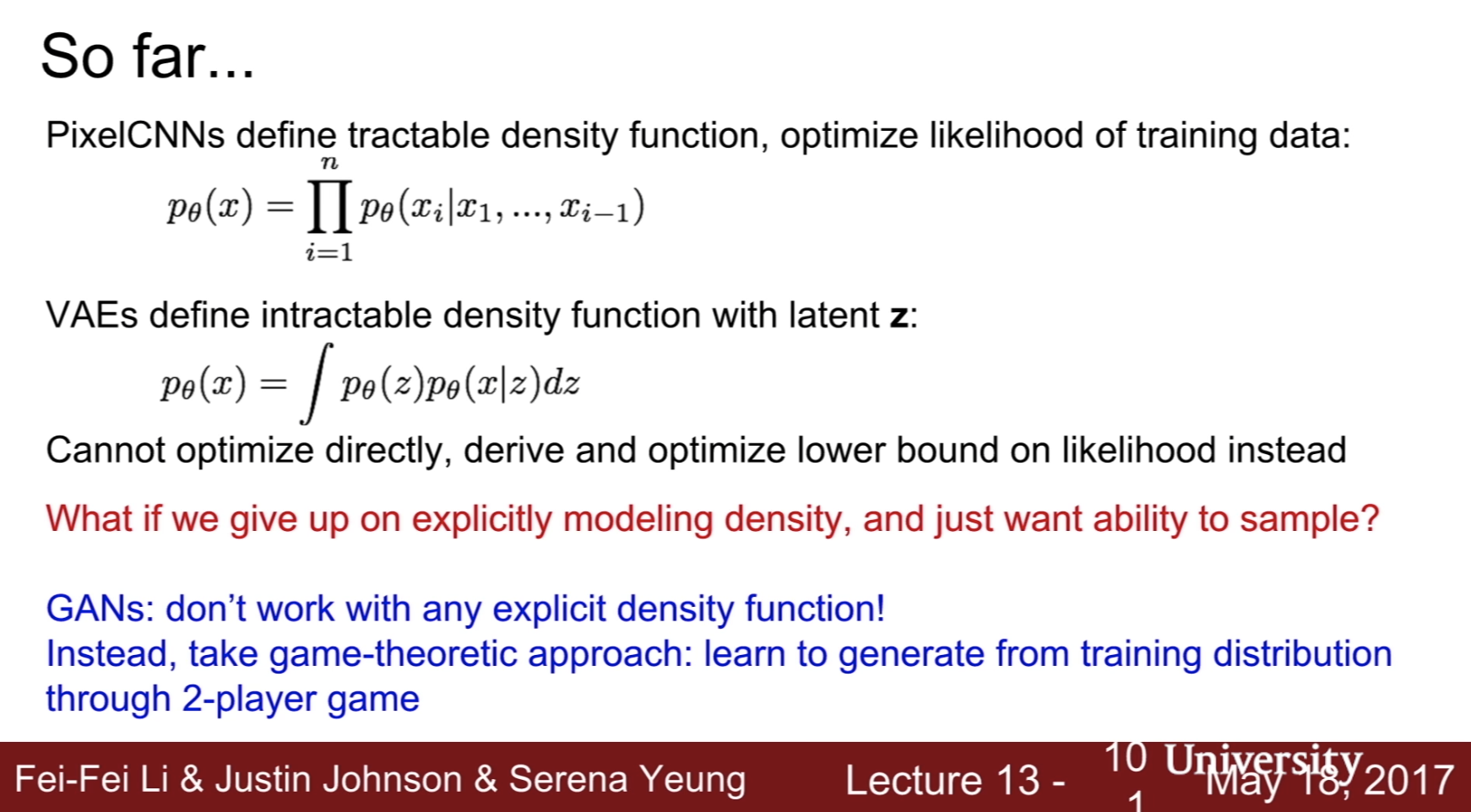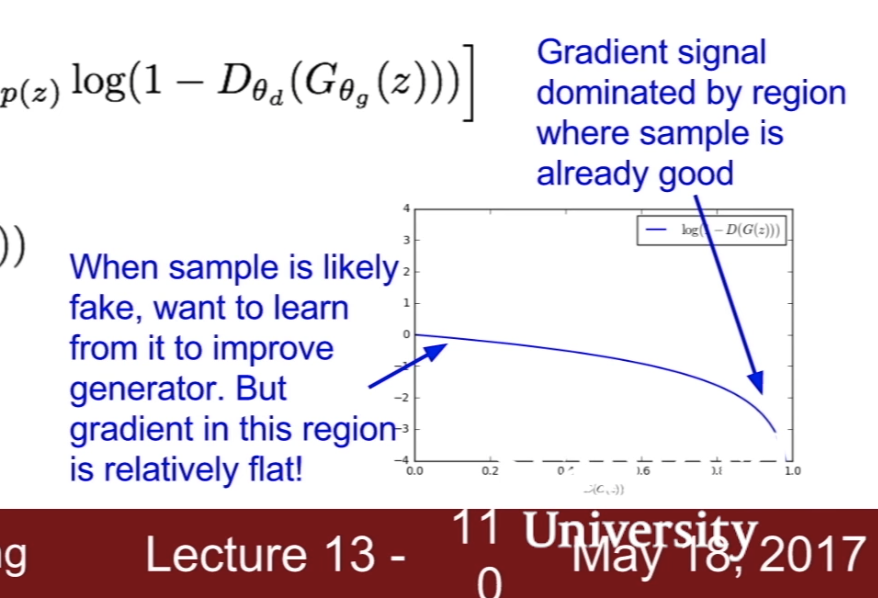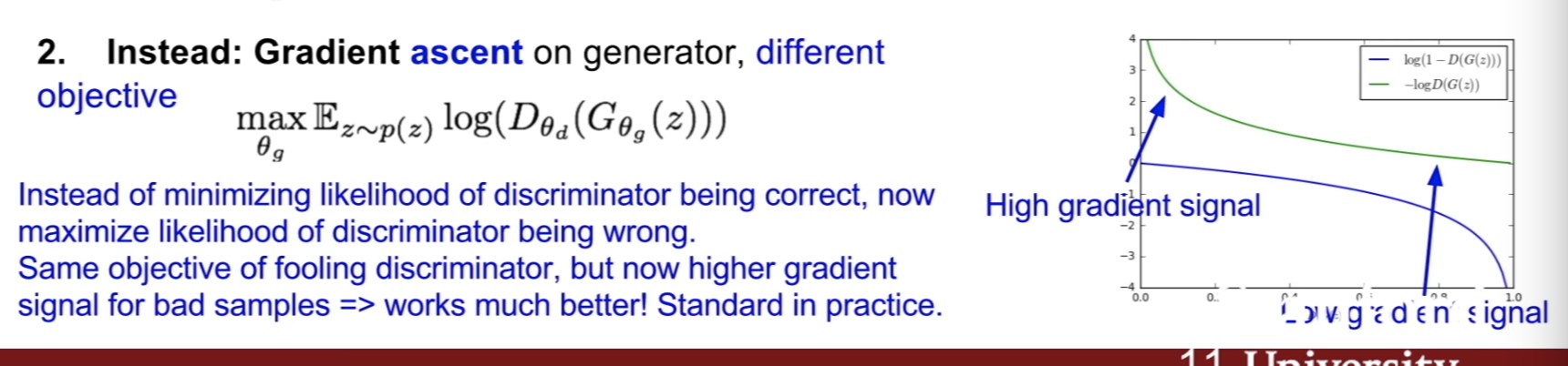Categories:

Updated: